keywords: KS statistics, lift, gain, area under receiver operating characteristic (ROC) curve, true postive rate, false positive rate, true negative rate, false negative rate, sensitivity, specificity, confusion/contingency matrix

# Introduction

A common way to evaluate the performance of a binary classifier is simply through overall accuracy or misclassification rate. But this is often not sufficient when the power of picking up the trues as true and/or picking up the falses as false is of interest. Examples of metrics that consider these true positive and true negative predictability power are area under receiver operating characteristic (AUROC) curve, Kolmogorov Smirnov (KS) statistics, Gini coefficients etc. In this post, we will see the definitions and how to calculate these metrics. Then we will give an overview of the gains table and discuss how to construct a gains table.

# Binary classifier and misclassification rate

A binary classifier is simply a classification model where the response has just two outcomes(Yes/No, 1/0, True/False, Male/Female, Good/Bad etc). A binary classifier can be made via logistic regression, regression tree, random forest, discriminant analysis, neural network, support vector machine and such. A good model, rather giving a straight forward decision (good/bad, 1/0), gives a probability being good or bad. One must decide a cutoff to label the predictions as good/bad or 1/0.

# Data set

For this demonstration, we will work with the mictrotus data from Flury package. While this is an ideal data for demonstrating multocollinearity, we will use the data to demostrate metrics of binary classifier.

There are in total 288 observations, each representing a vole. 43 of them are classified as multiplex and 46 are classified as subterraneus. The rest are unknown. We will work with the first 80 observations for simplicity. For simplicity, and for ease of calculations, let us code the two classes as 1 and 0.

library(Flury)
data("microtus")
mdata=microtus[c(1:80),c(1,2, 5)] ## read only first 80, keep only three variables
## code 1 and 0
mdata$Group=ifelse(mdata$Group=="multiplex", 1, 0)
summary(mdata)
##      Group            M1Left        Foramen
##  Min.   :0.0000   Min.   :1640   Min.   :3490
##  1st Qu.:0.0000   1st Qu.:1772   1st Qu.:3791
##  Median :1.0000   Median :1896   Median :3958
##  Mean   :0.5375   Mean   :1926   Mean   :3948
##  3rd Qu.:1.0000   3rd Qu.:2070   3rd Qu.:4097
##  Max.   :1.0000   Max.   :2479   Max.   :4662

The aim of this post is not covering all the classifiers. So we will work with simple logistic regression. Now from several class project I know that many predictors are correlated. Using only two predictors gives a reasonably good model. So only two predictors were kept in the earlier step. Let us make a logistic model for our chosen data.

model=glm(Group~M1Left+Foramen, data=mdata, family = binomial())
summary(model$fitted.values) ## Min. 1st Qu. Median Mean 3rd Qu. Max. ## 0.0000871 0.0115191 0.6049780 0.5375000 0.9997978 1.0000000 Now what this model gives us, a bunch of values in between 1 and 0. These are actually probabilities of being the class coded with 1 (multiplex). Now, to find an overall accuracy or error rate, one must choose the cutoff value. 0.5 seems an intuitive and obvious choice for the cut off. Let us use this cut off and find the error rate: ## a function for error rate get_Error_Rate=function(trues, predicted_prb, cutoff){ preds=ifelse(predicted_prb<cutoff,0,1) tab=table(preds, trues) round((tab[1,2]+tab[2,1])/sum(tab), 4) } get_Error_Rate(mdata$Group,model$fitted.values, 0.5) ##  0.05 So, using 0.5 as cutoff gives us a 5% error rate. But how to choose the cutoff? One potential solution is get error rate at every possible cutoff and choose one gives the lowest error rate. ## [,1] [,2] [,3] [,4] [,5] [,6] [,7] [,8] [,9] [,10] ## cutoff_value 0.1 0.15 0.2 0.25 0.3 0.3500 0.4000 0.45 0.50 0.5500 ## errRate 0.1 0.10 0.1 0.10 0.1 0.0875 0.0625 0.05 0.05 0.0625 ## [,11] [,12] [,13] [,14] [,15] [,16] [,17] ## cutoff_value 0.6000 0.65 0.70 0.75 0.80 0.8500 0.900 ## errRate 0.0375 0.05 0.05 0.05 0.05 0.0625 0.075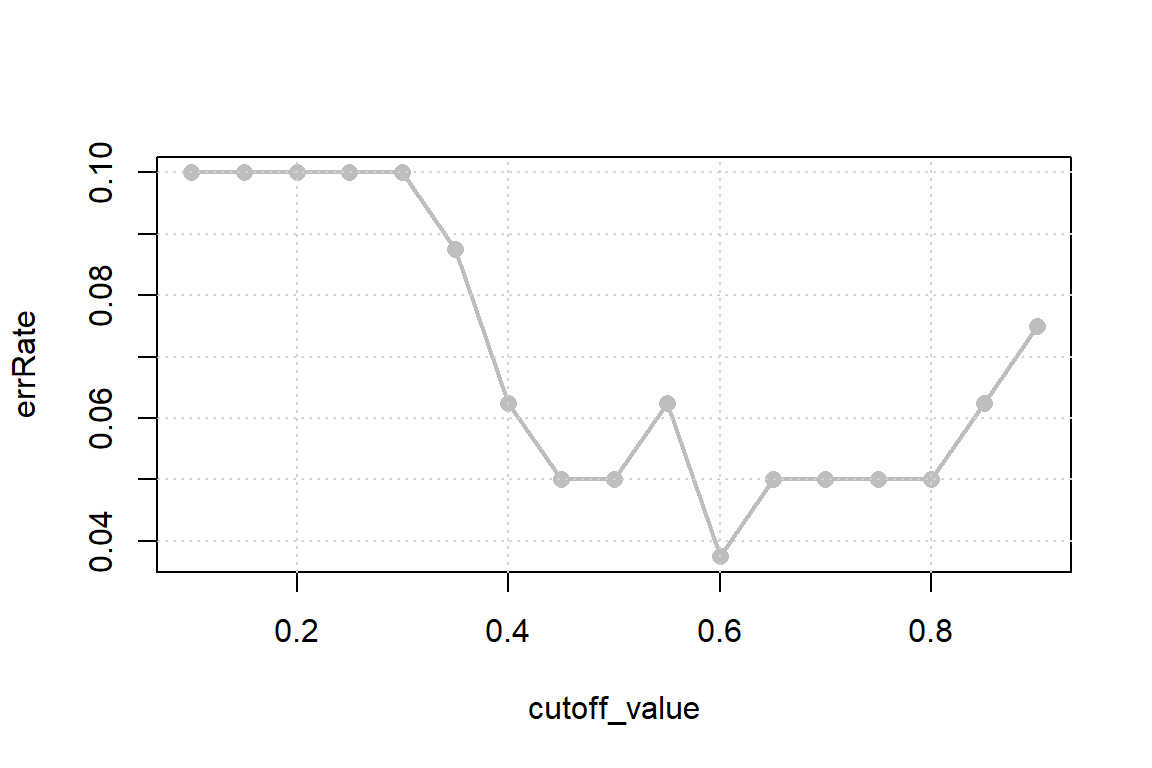Above figure shows that using 0.6 as cutoff actually gives a better overall accuracy. So using this value as cutoff is tempting. Now one problem associated with the overall error rate is that it does not account anything about the power of picking trues as trues and falses as false. In certain cases true positive rate might be of more interest than the overall error rate. To consider the power of predicting trues as true and falses as false, we need different metric. Two such metrics and AUROC and KS statisitc. # Area under ROC (AUROC) curve To get to the ROC curve, let us discuss the true positive rate, true negative rate, false positive rate and false negative rate first. These metrics really depend on what we define as positive and what we define as negative. For the current example, let us define code 1 as positive. ## True Postive Rate (TPR) It is the probability that the model detects an actual positve as postive. In other words, it is the proportion of the actual postives that are detected as positive by the model. This is also known as sensitivity. Mathematically: $TPR=P(\hat{x}=1|x=1)$ ## True Negative Rate (TNR) It is the probability that the model detects an actual negative as negative. In other words, it is the proportion of the actual negatives that are detected as negative by the model. This is also known as specificity. Mathematically: $TNR=P(\hat{x}=0|x=0)$ ## False Postive Rate (FPR) It is the probability that the model detects an actual negative as postive. In other words, it is the proportion of the actual negatives that are detected as positive by the model. This is also known as Type I error. Mathematically: $FPR=P(\hat{x}=1|x=0)$ ## False Negative Rate (FNR) It is the probability that the model detects an actual positive as negative. In other words, it is the proportion of the actual negatives that are detected as positive by the model. This is also known as Type II error. Mathematically: $FNR=P(\hat{x}=0|x=1)$ One good way to present the numbers is confusion matrix/contigency table. It shows the number of true postives, true negatives, false postives and false negatives. One can calculate TRP, FPR, TNR, FNR from the confusion matrix. createConfusionMatrix=function(actual, preds, cutoff_value){ predClass=ifelse(preds<cutoff_value, 0, 1) message("Cutoff is : ", cutoff_value) table(actual,predClass) } ## Confusion matrix for cutoff=0.5 createConfusionMatrix(mdata$Group,model$fitted.values, 0.5) ## Cutoff is : 0.5 ## predClass ## actual 0 1 ## 0 35 2 ## 1 2 41 The confusion matrix shown above is for cutoff=0.5. Here, $TP=35,\ TN=41, \ FP=2, \ FN=2$ These numbers are sensitive to the cutoff used. For example, for cutoff 0.8, the confusion matirx is shown below. Here the FPR decreased, while the overall error rate remained same. createConfusionMatrix(mdata$Group,model$fitted.values, 0.8) ## Cutoff is : 0.8 ## predClass ## actual 0 1 ## 0 37 0 ## 1 4 39 A good model aims to icrease the TPR while decreasing the FPR. But these two change in opposite direction. For example, if all the observations are predicted to be positive then TPR is 100%, which is good. But on the other hand, FPR is also 100% which is not desirable. Similarly if all the observations are predicted to be negative, then both FPR and TPR becomes 0%. So using alone TPR or TNR does not seem very intuitive. So need other measure that cares about both. ## ROC curve Now that TPR and FPR changes as does cut off, one can calculate a bunch of TPR and FPR for different cutoff values. Then if we plot the TPR along y-axis and FPR along x-axis then resulting curve is known as ROC curve. For our case, let us get the ROC curve. getTPRFPR=function(actual, predProb, cutoff){ if(cutoff==1){tpr=0; fpr=0}else{ if(cutoff==0){tpr=1; fpr=1}else{ predClass=ifelse(predProb<cutoff,0,1); tab=table(actual, predClass) tpr=tab[2,2]/ ( tab[2,2]+tab[2,1] ) tnr=tab[1,1]/ ( tab[1,1]+tab[1,2] ); fpr=1-tnr } } return(c(TPR=tpr, FPR=fpr)) } cutoff_values=seq(0,1,0.05)->TPR->FPR for(i in 1: length(TPR)){ metrics=getTPRFPR(mdata$Group,model$fitted.values,cutoff_values[i]) TPR[i]=metrics FPR[i]=metrics } head(cbind(cutoff_values, TPR, FPR)) ## cutoff_values TPR FPR ## [1,] 0.00 1.0000000 1.0000000 ## [2,] 0.05 1.0000000 0.2972973 ## [3,] 0.10 0.9767442 0.1891892 ## [4,] 0.15 0.9767442 0.1891892 ## [5,] 0.20 0.9534884 0.1621622 ## [6,] 0.25 0.9534884 0.1621622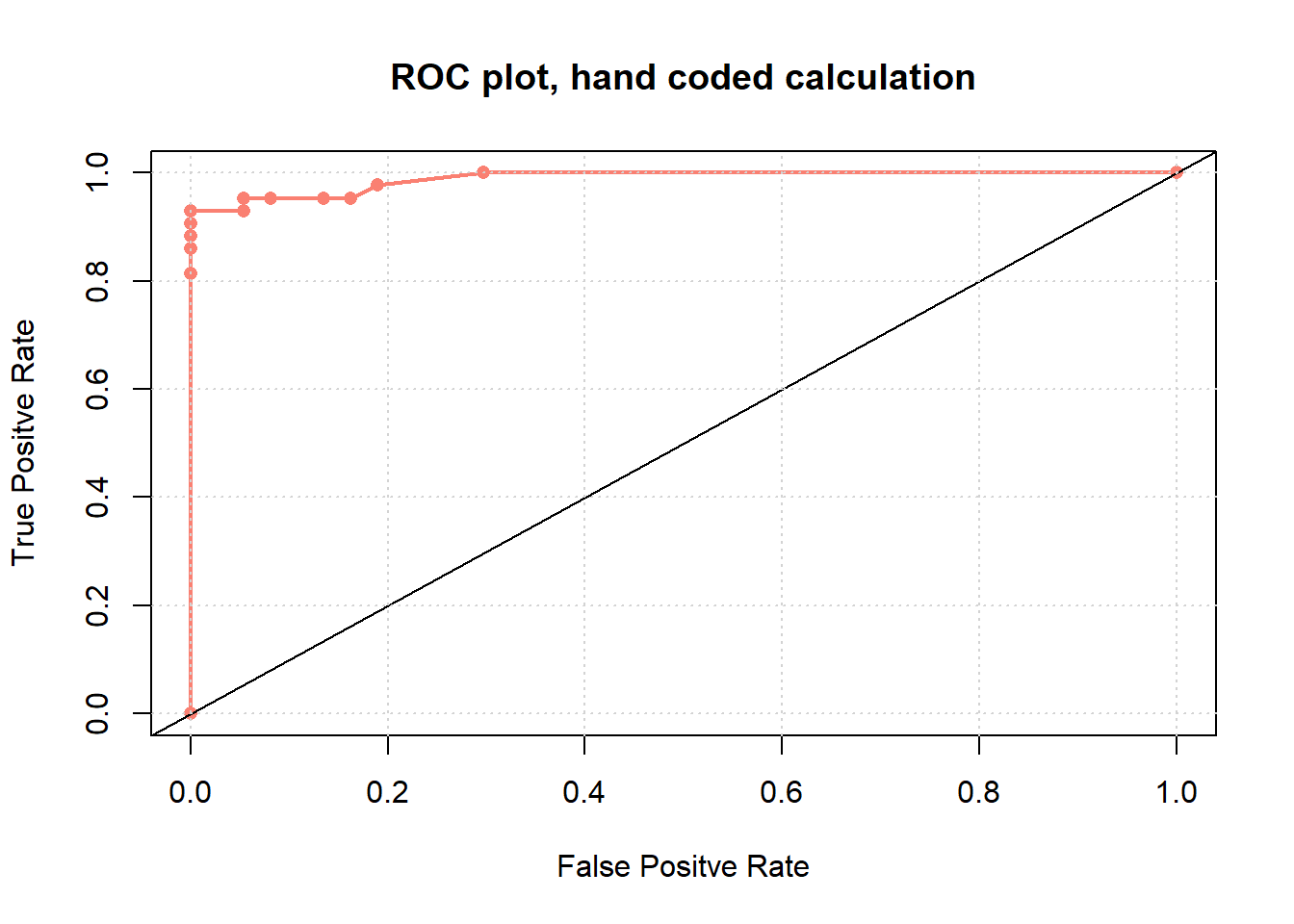Above we plotted the ROC curve. The black line represents the ROC if the model predicted randomly. It is not hard to say that one would like to pull the curve northwest as this indicates high TPR and low FPR. The area under this curve is called the AUROC. Ideally it can be maximum 1. The higher the value, the better it is. So, if we have two or more competitive models, AUROC can be used as a performance metric to choose one model. We manually generated the ROC chart above. But we can do this using ROCR package. library(ROCR) ## Warning: package 'ROCR' was built under R version 3.4.3 ## Loading required package: gplots ## ## Attaching package: 'gplots' ## The following object is masked from 'package:stats': ## ## lowess pred=prediction(model$fitted.values, mdata$Group) perf=performance(pred,"tpr","fpr") TPRfromROCR=unlist(perf@y.values) FPRfromROCR=unlist(perf@x.values) plot(TPRfromROCR~FPRfromROCR, col="deepskyblue", main="ROC plot- from ROCR package",type="o", pch=16);abline(0,1);grid()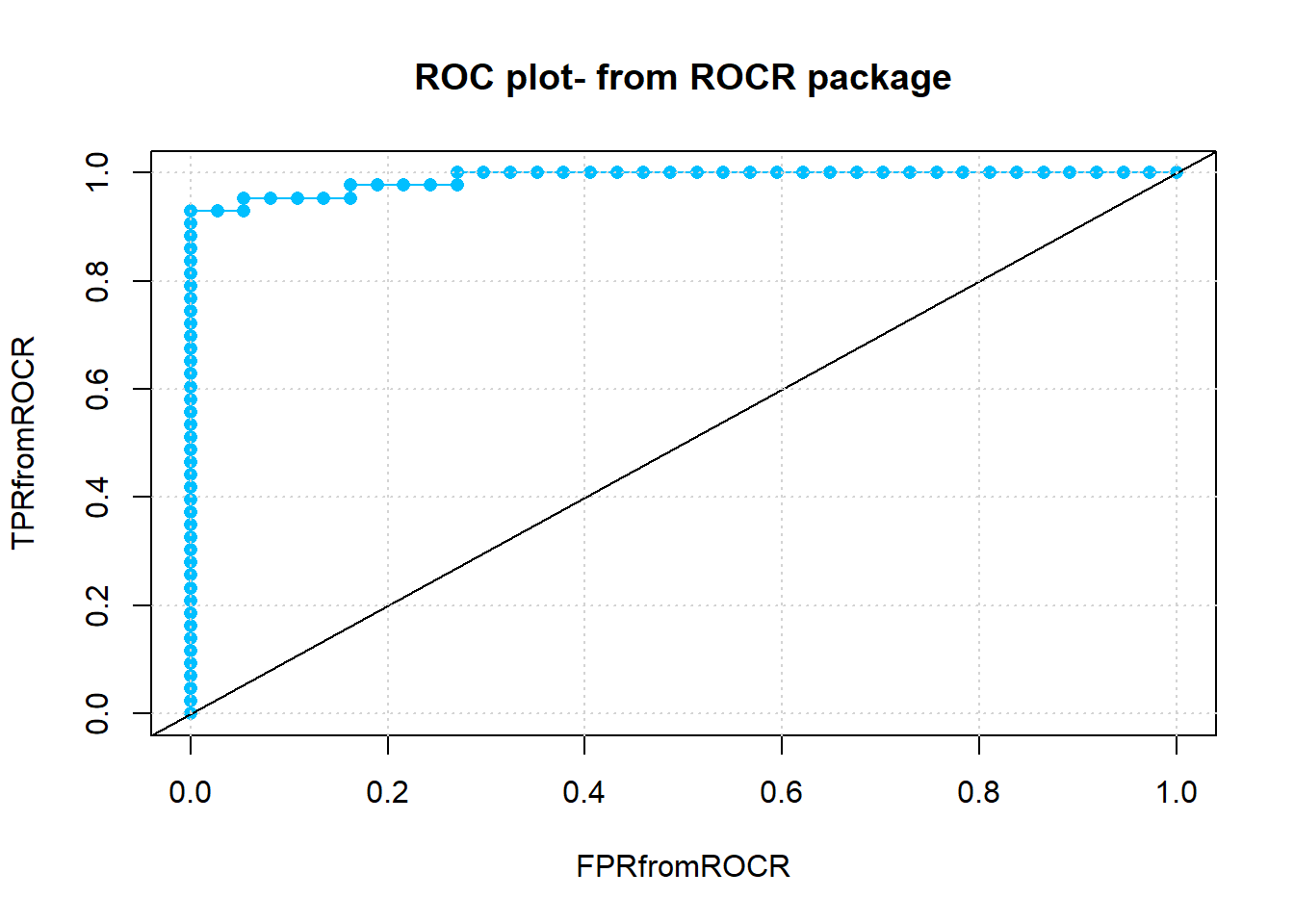## Get the AUC print(performance(pred,"auc")@y.values[]) ##  0.9886864 Above plot is made utilizing the ROCR package. We see that there are more cutoff points taken by the in built performance function. The AUROC is calcualted to be 0.99. This is actually very close to the ideal value. # KS statistic Along with AUROC, another measure is the KS statistic. It is the maximum difference between TPR and FPR. For our case, it is calculated to be 0.9302326, which is at cutoff = 0.6262503, diff_TPRFPR=TPRfromROCR-FPRfromROCR KS=max(diff_TPRFPR) cutoffAtKS=unlist(perf@alpha.values)[which.max(diff_TPRFPR)] print(c(KS, cutoffAtKS)) ##  0.9302326 0.6262503 In the figure below, a graphics of KS statistics is shown. Higher KS stat value is indicative of better model. If we have more than one models, then KS statistics can be used as a performance measure.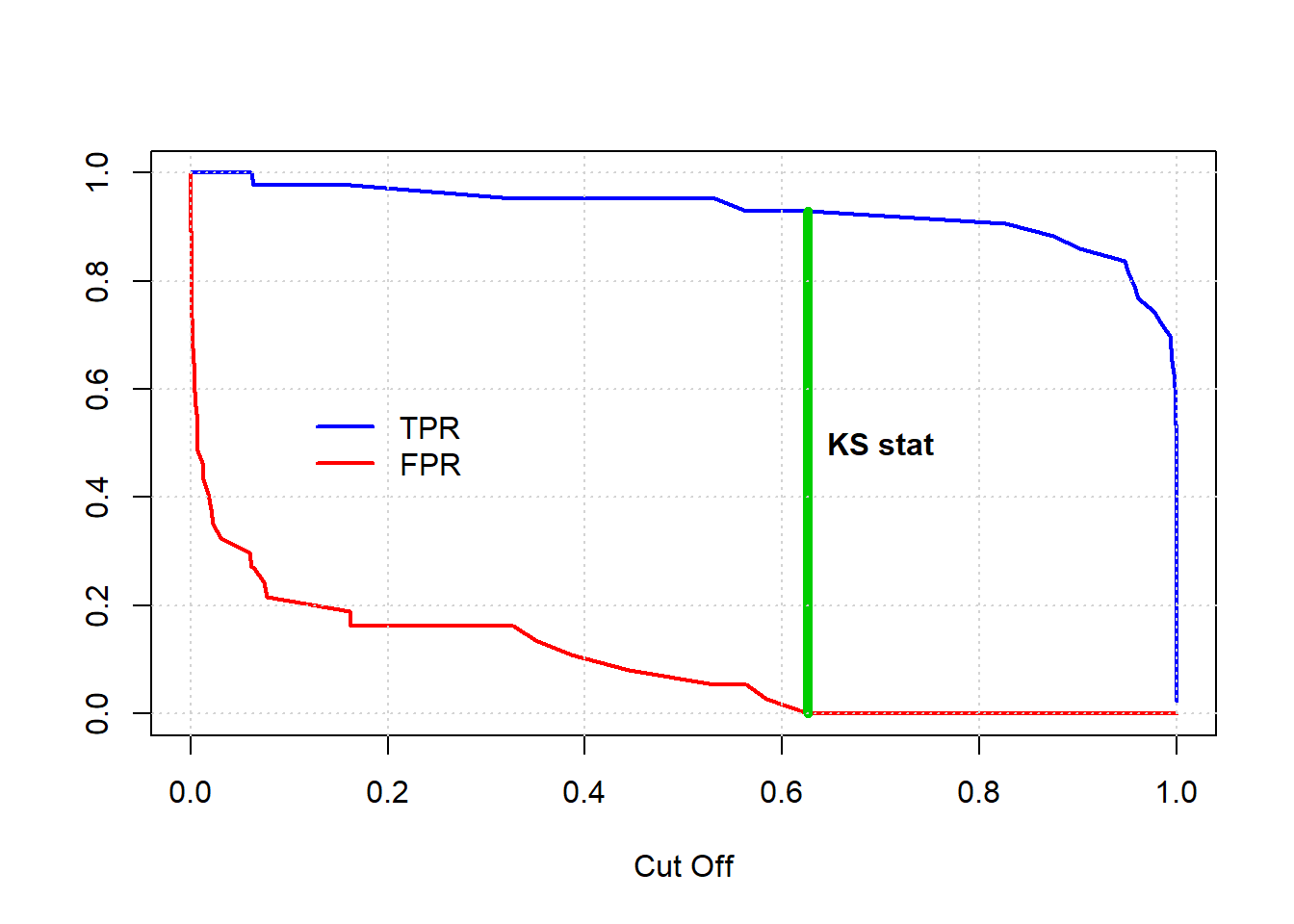The above figure for KS statistics can be shown in a different way. In the above figure, we took the cutoff along X-axis, and TPR and FPR along Y-axis. Instead we could take the population depth along X and proportion of 1s and 0s along Y. The max difference of two plots will be the KS stat. A more detailed explanation is given below: 1. From the model, we will have some probabilities of success. First, we need to sort the observations in descending order of these probabilities. 2. Now we will start with the first observation. This is almost guaranteed that the first observation will have an actual label 1. So for depth=1, the proporiton of success will be $$\frac{1}{number\ of \ total \ 1s}$$. And the proportion of failure would be $$\frac{0}{number\ of \ total \ 1s}$$. 3. Now for each possible depth, calculate the success and failure proportion. We have to keep in mind that this is cumulative proportion. So, at depth=n, success proportion would be $$\frac{number\ of\ 1s\ in\ first\ n\ rank\ ordered\ observation}{number\ of \ total \ 1s}$$ and failure proportion would be $$\frac{number\ of\ 0s\ in\ first\ n\ rank\ ordered\ observation}{number\ of \ total \ 1s}$$ 4. Now we will plot the cumulative success and failure proportion against depth and calculate the KS stat as the maximum difference of the two. Let us do it by hand. AppendedData=as.data.frame(cbind(actuals=mdata$Group, probs=model$fitted.values)) orderedData=AppendedData[order(AppendedData$probs, decreasing = T),]
totalOnes=sum(orderedData$actuals) totalZeros=nrow(orderedData)-sum(orderedData$actuals)
getprop=function(n){
myarray=orderedData$actuals[1:n] summyarray=sum(myarray) success_prop=summyarray/totalOnes failure_prop=(n-summyarray)/totalZeros return(c(success_prop, failure_prop)) } nroworder=nrow(orderedData) orderedData$cum_Success_Prop=rep(NA, nroworder)
orderedData$cum_Failure_Prop=rep(NA, nroworder) for(i in 0:nroworder){ k=getprop(i) orderedData$cum_Success_Prop[i]=k
orderedData$cum_Failure_Prop[i]=k } orderedData$Diff_Cum_Prop=orderedData$cum_Success_Prop-orderedData$cum_Failure_Prop
head(orderedData)
##    actuals     probs cum_Success_Prop cum_Failure_Prop Diff_Cum_Prop
## 26       1 1.0000000       0.02325581                0    0.02325581
## 11       1 1.0000000       0.04651163                0    0.04651163
## 17       1 0.9999995       0.06976744                0    0.06976744
## 5        1 0.9999989       0.09302326                0    0.09302326
## 14       1 0.9999987       0.11627907                0    0.11627907
## 42       1 0.9999958       0.13953488                0    0.13953488
message("The KS stat as follows")
## The KS stat as follows
max(orderedData$Diff_Cum_Prop) ##  0.9302326 We can see that the same KS stat is returned. We plotted the cumulative proportions against depth below. We see this plot has a different shape than the previous one. TPR and FPR started at 1 and ended at 0. In the later plot, the cumulative proportions started at zero and ended at 1. However, both has the same fundamental hysteresis-loop like shape. It is for the variable we are plotting along X, which is making the difference of the two plots.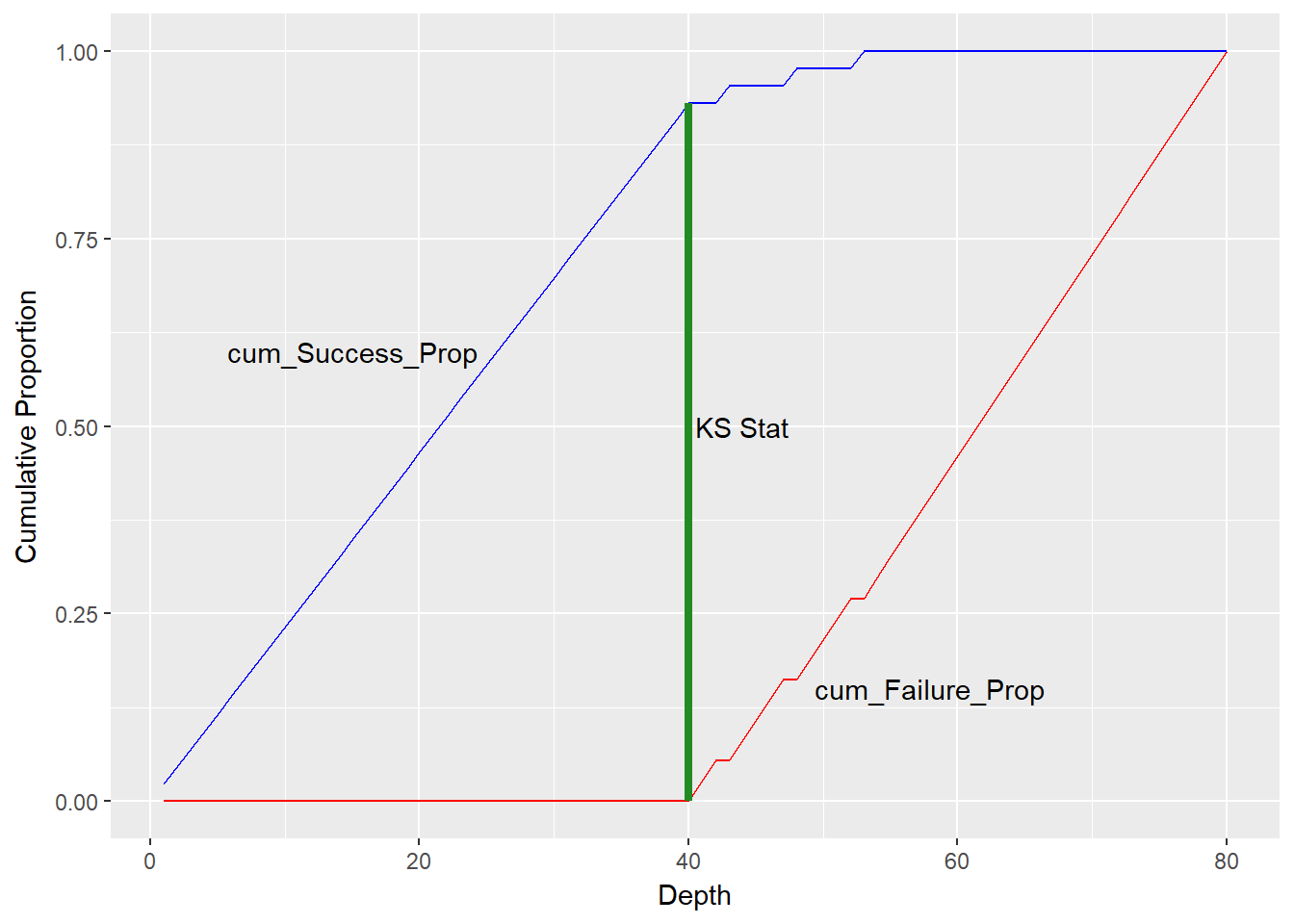# A different approach for preparing ROC curve To get the ROC curve, all that we need a set of TPR-FPR values at different settings. Previously we changed this setting by changing the cutoff. There is another approach, which is very efficient in a spread sheet. This requires to arrange the onbservation to be rank ordered (ordered by probability), just like we did to get the upward KS curve. The process is summarused as follows: 1. Rank order the observations, ordered by fitted/predicted probability. 2. Now for $$depth=n$$, treat first n predictions as success, the rest as failures. With these predictions, get TPR and FPR. 3. Find TPR and FPR for all possible depth. 4. Now we have a number of TPR-FPR pairs. Plot TPR against FPR. The resulting ROC curve will be same as before. Let us hand code what we discussed above.. :) All that we need just the original observations and the fitted/predicted probabilities. ## firsrt combine the actual class and fitted probabilities AppendedData=as.data.frame(cbind(actuals=mdata$Group, probs=model$fitted.values)) ## Rank order them, ordered by probs ndata=AppendedData[order(AppendedData$probs, decreasing = T),]

## Create two empty vectors to save TPR and FPR values
TPRvals=rep(NA, nrow(ndata))->FPRvals

## get number of total ones and zeros. we will need these
totalOnes=sum(ndata$actuals) nrows=nrow(ndata) totalZeros=nrows-sum(ndata$actuals)

## run loop
for(n in 1:nrows){
preds=c(rep(1,n), rep(0, (nrows-n))) ## first n predicted 1, rest are zero
TruePositive=sum(preds*ndata$actuals) ## retain only if both actual and pred 1 ## to get false positive ## we take only n ## multiply actuals and preds ## False postive will be zero ## this is sum-sum(product) FalsePositive=n-sum( (preds*ndata$actuals) [1:n])
TPRvals[n]=TruePositive/totalOnes
FPRvals[n]=FalsePositive/totalZeros
}

## Now plot the ROC
plot(TPRvals~FPRvals, type="o", col="#7CAE00", pch=16,
main="ROC plot, hand calculated by rank ordering"); abline(0,1, lwd=2, col="navyblue"); grid()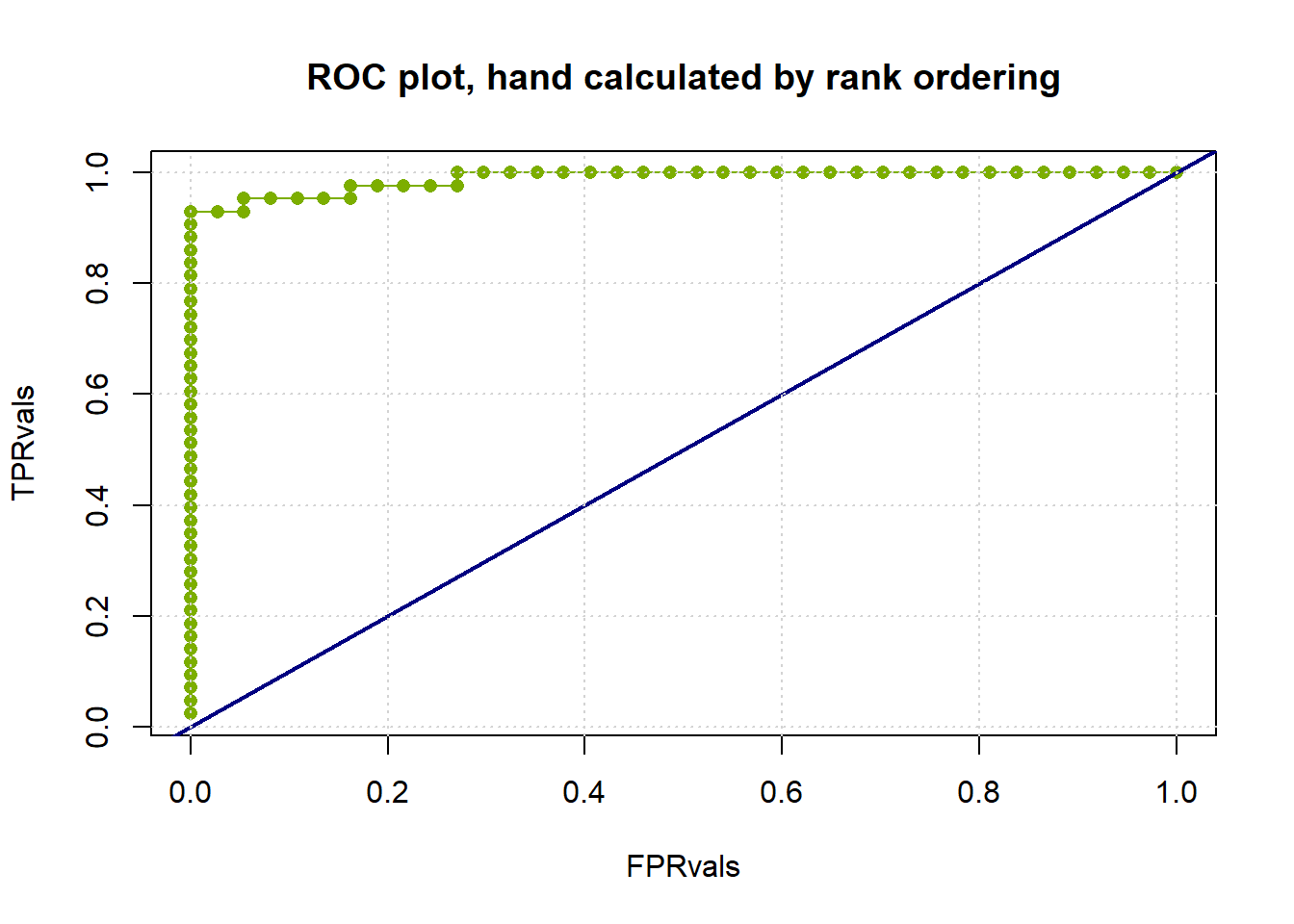Above ROC curve is exactly the same as we had before.

# Gini Coefficient

Gini coefficient is another measure of goodness of a binary classifier. The Gini coefficient is a ratio of two areas. The areas are:

1. The area between the ROC curve and the random model line ($$y=x$$ line, passing through $$(0,0)$$ and $$(1,1)$$)

2. The top left triangle above the random model line ($$y=x$$ line).

Now, the second area is just 0.5, since the domain and range of ROC are $$[0,1]$$. The first area is 0.5 less than the AUROC. So, Gini coefficient can be reduced to:

$Gini\ Coefficient=\frac{AUROC-0.5}{0.5}=\frac{AUROC}{0.5}-1=2\times AUROC-1$

So, Gini coefficient is just a scaled version of AUROC. So it is not a rocket science that, higher the Gini coefficient, better the model.

# Lift and Gain chart

• will complete later